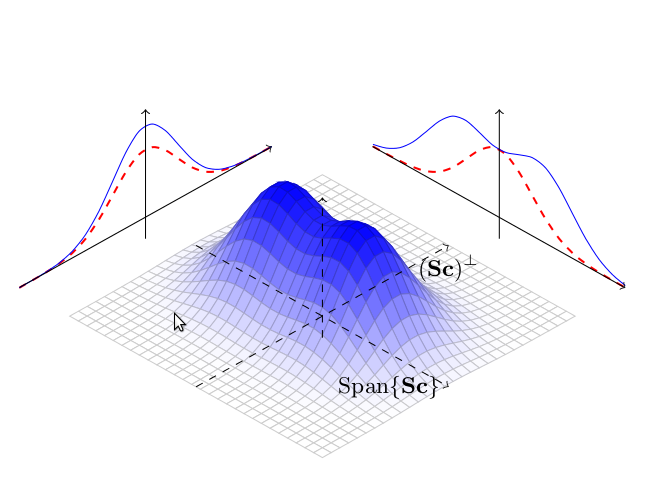Publications de Léo Ducas : Lattice Signatures and Bimodal Gaussians## Lattice Signatures and Bimodal Gaussians

Léo Ducas and Alain Durmus and Tancrède Lepoint and Vadim Lyubashevsky
Published at CRYPTO '13

Our main result is a construction of a lattice-based digital signature scheme that represents an improvement, both in theory and in practice, over today's most efficient lattice schemes. The novel scheme is obtained as a result of a modification of the rejection sampling algorithm that is at the heart of Lyubashevsky's signature scheme (Eurocrypt, 2012) and several other lattice primitives. Our new rejection sampling algorithm which samples from a bimodal Gaussian distribution, combined with a modified scheme instantiation, ends up reducing the standard deviation of the resulting signatures by a factor that is asymptotically square root in the security parameter. The implementations of our signature scheme for security levels of 128, 160, and 192 bits compare very favorably to existing schemes such as RSA and ECDSA in terms of efficiency. In addition, the new scheme has shorter signature and public key sizes than all previously proposed lattice signature schemes.
As part of our implementation, we also designed several novel algorithms which could be of independent interest. Of particular note, is a new algorithm for efficiently generating discrete Gaussian samples over Z^n. Current algorithms either require many high-precision floating point exponentiations or the storage of very large pre-computed tables, which makes them completely inappropriate for usage in constrained devices. Our sampling algorithm reduces the hard-coded table sizes from linear to logarithmic as compared to the time-optimal implementations, at the cost of being only a small factor slower.

Full version PDF
eprint: http://eprint.iacr.org/2013/383
Source Code: http://bliss.di.ens.fr/